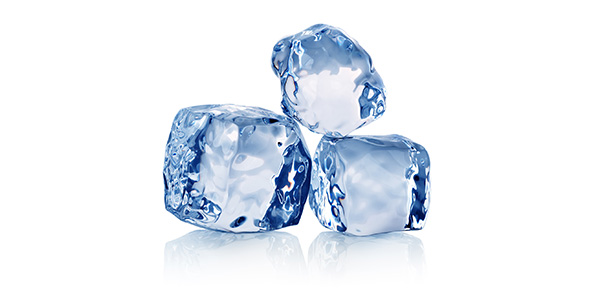# Glaciers Rivers Of Ice

5 Questions | Total Attempts: 71SettingsLets see if you were paying attention

• 1.
What is a Glacier
• A.

Small ice cubes in a punch bowl

• B.

A large, fast mass of moving ice

• C.

A small, slow mass of moving ice

• D.

A large, slow mass of moving ice

• 2.
Alpine Glaciers form in
• A.

Low elevations where it is hot

• B.

High elevations where temperatures remain cold

• C.

High elevations where temperatures remain mild

• D.

Low elevations where temperatures remain cold

• 3.
A continental glacier is
• A.

A huge mass of ice that covers a lot of land

• B.

A huge mass of ice that covers a city

• C.

A small mass of ice that does not cover anything

• D.

A small mass of ice that covers a state

• 4.
How many of the United States is the Ross Ice Shelf
• A.

One

• B.

Two

• C.

Seven

• D.

One and a half

• 5.
Where do Valley Glaciers form
• A.

In mountainus areas

• B.

In low areas

• C.

In rich people's backyards

• D.

By rivers

Related TopicsBack to top